# ChIPS - using symbols to draw sets of (x,y) pointsChIPS Threads (CIAO 4.11 ChIPS v1)

## Overview

#### Synopsis:

This thread is intended to give a simple demonstration of the support for symbols and error bars in ChIPS. It also includes examples of how ASCII files can be used in ChIPS (and in other CIAO tools), as well as how data can be plotted directly from a file or read into Python, manipulated, and then sent to ChIPS. Additional capabilities, such as annotating the plot with lines and labels, changing the order items are drawn, and use of multiple plots are included.

Last Update: 15 Dec 2015 - Updated for CIAO 4.8.

## Introduction

The Starting ChIPS thread describes how to start ChIPS. Please see the ChIPS GUI section of that thread for information on the ChIPS GUI.

The data used in this thread is available in the chips_data.tar.gz file.

unix% ls -1 chips/curve_symbol/
bax.lis


The plots used in this thread are based on the data in the text file bax.lis which contains the contents of the BAX Galaxy Cluster Database. The data was obtained from the VizieR catalogue service and converted into a form that could be recognized by the ASCII kernel of the CIAO Data Model.

The file consists of columns separated by the | character and looks like:

unix% head bax.lis
#name|ra|dec|z|fx|lx|tx|telo|tehi
MS1008.1-1224|152.6423|-12.6632|0.301|3.02e+00|1.10e+01|7.20|0.80|1.00
ABELL1656|194.9531|+27.9807|0.023|3.44e+02|7.77e+00|8.25|0.10|0.10
RXJ1120.1+4318|170.0312|+43.3014|0.600|4.20e-01|6.07e+00|5.45|0.30|0.30
RXJ1053.7+5735|163.4308|+57.5892|1.263|2.64e-02|1.92e+00|3.90|0.20|0.20
RXJ0123.6+3315|020.9167|+33.2556|0.016|1.71e+01|1.98e-01|||
ABELL3112|049.4683|-44.2429|0.075|3.10e+01|7.37e+00|4.72|0.15|0.22
FORNAXCLUSTER|054.6289|-35.4545|0.005|9.02e+01|8.16e-02|1.56|0.04|0.03
IIIZW054|055.3242|+15.3961|0.029|2.00e+01|7.19e-01|||
ABELL3158|055.6652|-53.6306|0.060|3.79e+01|5.65e+00|5.41|0.14|0.16
unix% dmlist "bax.lis[opt sep=|]" cols

--------------------------------------------------------------------------------
Columns for Table Block bax.lis
--------------------------------------------------------------------------------

ColNo  Name                 Unit        Type             Range
1   name                              String
2   ra                                Real8          -Inf:+Inf
3   dec                               Real8          -Inf:+Inf
4   z                                 Real8          -Inf:+Inf
5   fx                                Real8          -Inf:+Inf
6   lx                                Real8          -Inf:+Inf
7   tx                                Real8          -Inf:+Inf
8   telo                              Real8          -Inf:+Inf
9   tehi                              Real8          -Inf:+Inf


The [opt sep=|] text is needed to tell the Data Model that the column separator is | (the default value is white space). The column names are taken from the single comment line at the head of the file (which is also separated by | characters). The following example shows how the statistics of the redshift (i.e. z) column can be calculated using the dmstat tool:

unix% dmstat "bax.lis[cols z][opt sep=|]" sig-
z
min:        0.003         @:        1569
max:        1.301         @:        1133
mean:        0.16625139319
sum:        268.496
good:        1615
null:        98



## Symbol support for curves

In this section we are going to plot up the flux versus redshift values from the file bax.lis, varying the symbol used to mark the points to highlight the symbol support for curves in ChIPS. Since the file is not ordered by redshift we will turn off the lines connecting the points by setting the line.style attribute to none. The call to clear is not needed if ChIPS has just been started.

chips> clear()
chips> make_figure("bax.lis[cols z,fx][opt sep=|]")
chips> set_curve(["line.style", "none"])
chips> log_scale()


### Figure 1: Flux versus redshift using the default symbol style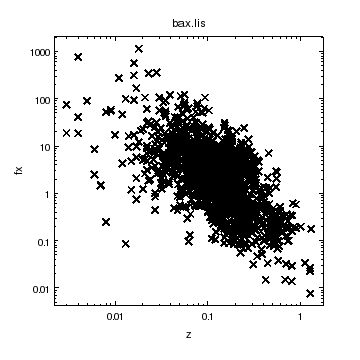### Figure 1: Flux versus redshift using the default symbol style

The make_figure command has created a plot of the fx versus z columns from the file bax.lis. The default symbol style (cross) is used to indicate the points; the line drawn between the points has been turned off by setting the line.style attribute to none.

ChIPS supports a range of symbol styles (Figure 19), such as a cross or a square, and these symbols can be drawn filled or open. The default values are to use a filled cross, as shown in Figure 1, and the following (Figure 2) show how the plot looks when an open square is used instead.

chips> set_curve(["symbol.style", "square", "symbol.fill", False])


### Figure 2: Flux versus redshift: open square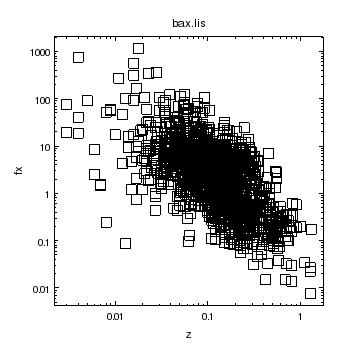### Figure 2: Flux versus redshift: open square

The symbol style has been changed to a square, and the symbol.fill attribute turned off.

The ChIPS GUI provides an easy way to inspect and modify your visualizations; an example of the GUI is shown in the 'Curves & Lines' thread and the properties editor is shown in Figure 16.

The symbol size can also be changed. The value used in Figure 2 is 5 (as returned by get_curve), with the valid range being 1 to 100, inclusive.

chips> get_curve().symbol.size
5
chips> set_curve(["symbol.size", 1])
chips> set_curve(["symbol.size", 2])


### Figure 3: Flux versus redshift: smaller squares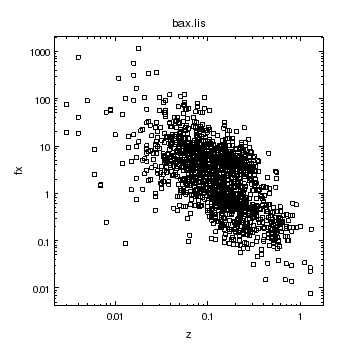### Figure 3: Flux versus redshift: smaller squares

The symbol size has been changed from its default value of 5, which was used in Figure 2, to 2.

The point symbol style is a special case in that its appearance is not changed by the symbol.fill and symbol.size attributes of the curve.

chips> set_curve(["symbol.style", "point"])


### Figure 4: Flux versus redshift: the point style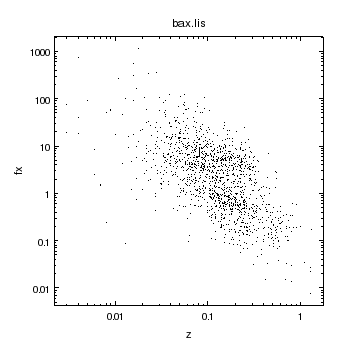### Figure 4: Flux versus redshift: the point style

The symbol style has been changed to point.

We add a second curve which consists of just the hot (Tx > 6 keV) clusters. A Data Model filter is used to select the points to plot, and the ChipsCurve object is used to set the attributes of the curve to make the new curve appear as red points.

chips> ci = ChipsCurve()
chips> ci.symbol.style = "point"
chips> ci.symbol.color = "red"
chips> ci.line.style = "none"


### Figure 5: Flux versus redshift: highlighting a subset of points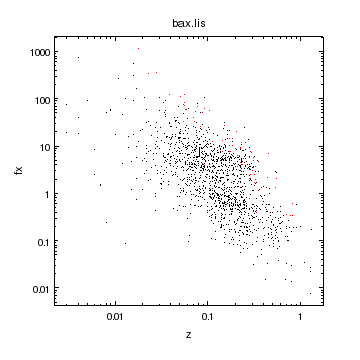### Figure 5: Flux versus redshift: highlighting a subset of points

A second curve has been added to the plot. This curve represents the hot (Tx > 6 keV) clusters and is drawn using red points.

In order to make these points more visible we change the symbol style to a small square rather than a point:

chips> ci.symbol.style = "square"
chips> ci.symbol.size = 2
chips> set_curve(ci)


The plot is completed by adding annotations to help identify the samples. Note that the valign attribute of the labels is set to 0.5 so that they are vertically centered on the associated point. A line has been used to draw a rectangle around the text and points (undo is useful here when trying to adjust the spacing of the box around the contents).

chips> add_point(0.2, 600, ["style", "point"])
chips> add_point(0.2, 300, ["style", "square", "size", 2, "color", "red"])
chips> add_label(0.23, 600, r"kT_x \leq 6 keV", ["valign", 0.5])
chips> add_label(0.23, 300, "kT_x > 6 keV", ["valign", 0.5, "color", "red"])
chips> xr = [0.16, 0.9, 0.9, 0.16, 0.16]
chips> yr = [170, 170, 900, 900, 170]
chips> print_window("plot-z-fx.eps")
chips> print_window("plot-z-fx.png")
chips> save_state("plot-z-fx.state")


### Figure 6: Flux versus redshift: the final plot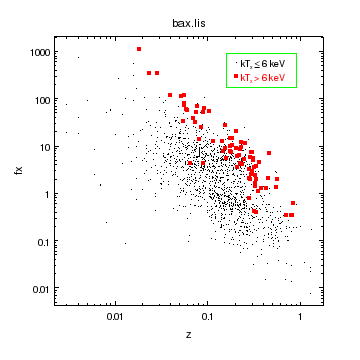### Figure 6: Flux versus redshift: the final plot

The second curve shown in Figure 5 has been removed and replaced by one which uses small red squares, since this is more visible. Two labels have been added to indicate what the curves are, and a line has been used to draw a rectangle around these labels.

## Handling data from a file

In this section we shall read in the data from bax.lis into Python using Crates, show how ChIPS handles missing values (e.g. NaN), and use a region to highlight a portion of the plot.

First we read in the file using read_file, and extract the data for the redshift (z) and temperature (tx) columns. The name column is removed just to avoid warning messages from get_col_names (or save_state), as it clashes with an existing attribute of a Crate (namely the "name" attribute which stores the file name).

chips> cr = read_file("bax.lis[opt sep=|][cols -name]")
chips> print(get_col_names(cr))
['ra', 'dec', 'z', 'fx', 'lx', 'tx', 'telo', 'tehi']
chips> z = copy_colvals(cr, "z")
chips> tx = copy_colvals(cr, "tx")
chips> z.size
1713
chips> np.isnan(z).sum()
98
chips> np.isnan(tx).sum()
1403
chips> np.isnan(z*tx).sum()
1407


Although the file contains 1713 entries, 98 of them do not contain redshift information, 1403 do not contain tx values, and 1407 do not contain either a redshift or tx value. The missing data items are recorded as NaN values in the arrays; as we shall see, ChIPS will ignore these values when creating curves.

We now use add_curve to plot these points using the open symbol style. To avoid having to turn the line style to none for any new curves we set the preference value for this attribute.

chips> clear()
chips> set_preference("curve.line.style", "none")
chips> add_curve(z, tx, ["symbol.style", "circle", "symbol.fill", False])
chips> log_scale()


### Figure 7: Temperature versus redshift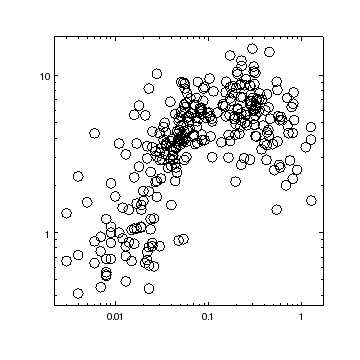### Figure 7: Temperature versus redshift

The temperature versus redshift arrays have been plotted as open circles, after being read in from the data file. Points which have contain a NaN value for either the redshift or temperature are ignored by ChIPS when creating the plot.

An alternate method is to filter out the unwanted points before plotting. When creating Figure 5 we used a Data Model filter to exclude cool clusters. Now we shall use the array-filtering capabilities of Python to exclude those entries which do not have valid data:

chips> i = np.isnan(z*tx) == False
chips> z2 = z[i]
chips> tx2 = tx[i]


The z2 and tx2 arrays can be used to create a plot:

chips> clear()
chips> add_curve(z2, tx2, ["symbol.style", "circle", "symbol.fill", False])
chips> log_scale()
chips> set_plot_xlabel("z")
chips> set_plot_ylabel("T_x")


We add on a horizontal line at Tx = 2, drawn with a dotted line style. A region is also added to the plot in order to highlight the redshift range z=0.2 - 0.9. The use of get_plot_yrange is to ensure that the region covers the full Y axis, and the fill.style attribute is set so that the interior of the region is filled with a solid color. The default fill.color for regions is green and the opacity is 0.5, which means that the on-screen version of the plot will show the points that lie behind the region.

The only formats not to support opacity (of regions and histograms) or alpha values (images) are postscript and encapsulated-postscript; compare the hardcopy versions of Figure 8.

chips> add_hline(2, ["style", "dot"])
chips> yr = get_plot_yrange()
chips> xbox = [0.2, 0.9, 0.9, 0.2]
chips> ybox = [yr, yr, yr, yr]
chips> print(get_region())
angle = 0.0
depth = 100
edge.color = green
edge.style = 1
edge.thickness = 1.0
fill.color = green
fill.style = 1
id = None
opacity = 0.5
stem = None

chips> print_window("z-tx-region-front")


### Figure 8: Temperature versus redshift: using a filled region to highlight a band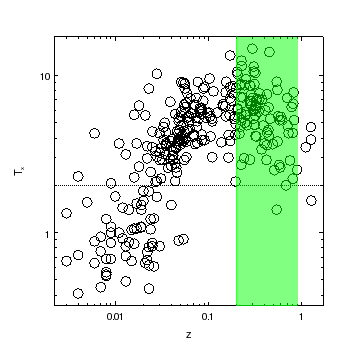### Figure 8: Temperature versus redshift: using a filled region to highlight a band

The region is drawn in front of the curve, so those symbols that fall in the region z=0.2 - 0.9 are visible, but tinted green, in the on-screen version of the plot and are hidden in the PS version of the plot created by the print_window command.

In order to make the symbols visible in the PS version of the plot we move the region to a lower depth than the curve. This means that the region gets drawn before the curve (and everything else, which has the same depth value as the curve), and so will not obscure the symbols.

chips> get_region().depth
100
chips> get_curve().depth
100
chips> set_region(["depth", 50])
chips> print_window("z-tx-region-back")
chips> save_state("z-tx-region-back.state")


### Figure 9: Temperature versus redshift: moving the region behind the curve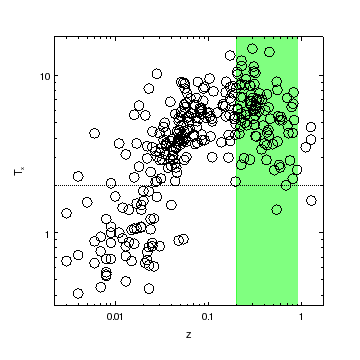### Figure 9: Temperature versus redshift: moving the region behind the curve

As the region has been moved to a lower depth than the curve (and all the other elements of the plot), it is drawn first, and so does not obscure the other elements in the PS version of the plot. This can be seen by comparing the postscript output of this figure to that of Figure 8.

The relative order that objects are drawn can also be changed by selecting an object with the mouse and then using the Shuffle Object iem from the right-mouse-button menu in the ChIPS window.

The fill.style attribute can be changed to alter the appearance of the region, as shown in Figure 10:

chips> set_region(["fill.style", "crisscross", "edge.style", "none"])


### Figure 10: Temperature versus redshift: using a crisscross pattern for the region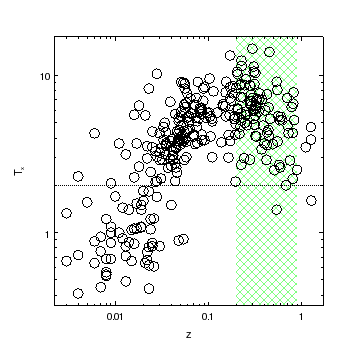### Figure 10: Temperature versus redshift: using a crisscross pattern for the region

The region is now filled with a green criss cross pattern rather than a solid fill.

## Error bars and limits

So far all the data we have plotted has not included error bars. As the BAX database includes errors for the temperature values (given as asymmetric values using the telo and tehi columns), we can use the data to highlight the support for error bars in ChIPS.

For the first plot we will assume symmetric error bars, so we decide to use the average value of the upper and lower error bars for illustrative purposes only. Since we want to exclude the values without temperatures or temperature errors, we filter out these values (and create the index array j):

chips> txlo = copy_colvals(cr, "telo")
chips> txhi = copy_colvals(cr, "tehi")
chips> txlo2 = txlo[i]
chips> txhi2 = txhi[i]
chips> dtx2 = 0.5 * (txlo2 + txhi2)
chips> j = numpy.isnan(dtx2) == False


The data can be plotted: if add_curve is given three 1D columns of data then it assumes they are x,y,dy values. The axis scale can be changed between linear and logarithmic scales, even when the data includes error bars. The resulting plot is shown in Figure 11.

chips> clear()
chips> log_scale()
chips> set_curve(["symbol.style", "square", "symbol.fill", False, "symbol.size", 2])
chips> set_plot_xlabel("redshift")
chips> set_plot_ylabel("Temperature (keV)")
chips> set_plot_title("BAX clusters with temperature errors")


### Figure 11: Temperature versus redshift: symmetric errors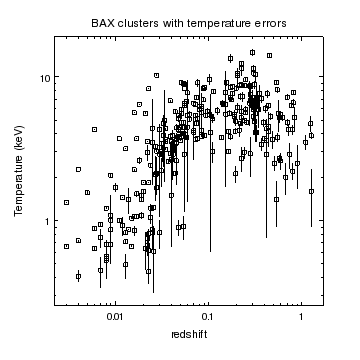### Figure 11: Temperature versus redshift: symmetric errors

Symmetric error bars have been drawn for the temperature measurements. These errors were calculated as the average of the upper and lower error bars for the data and so are only for illustrative purposes.

If the third argument to add_curve is not a 1D vector but a list of 1D vectors, then the values of the third argument are taken to be one of:

• low y error, high y error
• low y error, high y error, low x error
• low y error, high y error, low x error, high x error

We can therefore plot the asymmetric temperature errors using the following (where we re-use the index array j to exclude points with no error data). The error-bar style is changed from the default value of bar - as used in Figure 11 - to cap, and the colors of the error bars and symbols are also changed. The plot title is changed to be aligned to the left of the plot - by setting both title.halign and title.xpos to 0 and to use the times font.

chips> clear()
chips> set_curve(["symbol.style", "diamond", "symbol.fill", False, "symbol.size", 4])
chips> set_curve(["err.style", "cap", "err.color", "red"])
chips> set_curve(["symbol.color", "skyblue"])
chips> set_plot_title ("Asymmetric error bars")
chips> set_plot(["title.halign", 0, "title.xpos", 0, "title.font", "times"])
chips> log_scale()
chips> save_state("z-tx-error2.state")


### Figure 12: Temperature versus redshift: asymmetric errors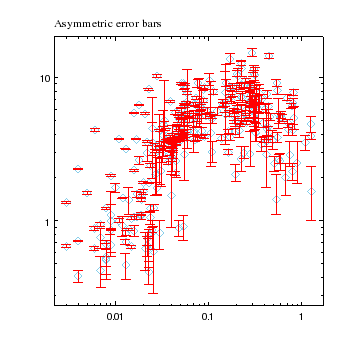### Figure 12: Temperature versus redshift: asymmetric errors

Asymmetric error bars have been drawn for the temperature measurements. The properties of the curve (error bar style, color, and symbol color) have been changed from their default values. Similarly, the plot title has been adjusted so that it is left-aligned with the plot and uses a different font than normal (times rather than helvetica).

The following show the settings of some of the elements of the figure - the symbol and error settings of the curve and the title settings of the plot.

chips> print(get_curve().symbol)
angle = 0.0
color = skyblue
fill = False
size = 4
style = 2

chips> print(get_curve().err)
caplength = 10
color = red
down = True
left = False
right = False
style = capped
thickness = 1.0
up = True

chips> print(get_plot().title)
angle = 0.0
color = default
depth = 100
font = times
fontstyle = normal
halign = 0
size = 16
valign = 0.5
xpos = 0
ypos = 15.0



In order to show error bars on the X axis we are going to use some invented data: y = sqrt(x) where the fractional error on y is 10% and the absolute error on x is 0.1. We set up the a, da, b, and db arrays with these values:

chips> a = np.arange(1,10,0.5)
chips> da = a * 0 + 0.1
chips> b = np.sqrt(a)
chips> db = b / 10.0


Now we can plot the data. We first create two plots, arranged vertically, using split, and then change the currency so that both plots are current. This means that the add_curve call creates curves in both plots. The second plot is selected (made "current") so that the set_curve call only changes the error-bar style of the curve in the bottom plot.

chips> clear()
chips> split(2)
chips> print(info())
Window [win1]
Frame [frm1]
Plot [plot1]   (0.15,0.52)  .. (0.90,0.90)
Border bottom [bx1]  top [bx2]  left [by1]  right [by2]
Plot [plot2]   (0.15,0.15)  .. (0.90,0.52)
Border bottom [bx1]  top [bx2]  left [by1]  right [by2]

chips> print(info_current())
Window [win1]
Frame [frm1]
Plot [plot1]
Coord Sys [Plot Normalized]

chips> current_plot("all")
chips> print(info_current())
Window [win1]
Frame [frm1]
Plot [plot1]
Plot [plot2]

chips> add_curve(a, b, [db, db, da, da], ["symbol.style", "none"])
chips> print(info_current())
Window [win1]
Frame [frm1]
Plot [plot1]
Curve [crv1]
X Axis [ax1]
Y Axis [ay1]
Plot [plot2]
Curve [crv1]
X Axis [ax1]
Y Axis [ay1]

chips> current_plot("plot2")
chips> print(info_current())
Window [win1]
Frame [frm1]
Plot [plot2]
Curve [crv1]
X Axis [ax1]
Y Axis [ay1]
Coord Sys [Data]
Coord Sys ID [ds5.5.6.27]

chips> set_curve(["err.style", "cap"])


Note that although the two curves were created by a single call, they are independent objects once they have been created, as shown by the fact you can change the err.style attribute of the curve in the second plot, as shown in Figure 13.

### Figure 13: Error bars on both X and Y values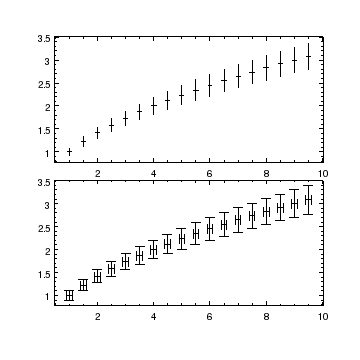### Figure 13: Error bars on both X and Y values

The two plots show the same data: y = sqrt(x) with 10% errors on y and an absolute x error of 0.1. The only difference in the two plots is that the err.style of the curve in the top plot has the default value of bar, whereas for the lower plot the curve's attribute has been changed to cap.

We finish this section with a brief discussion for the preliminary support of limits in ChIPS. Limits are indicated by supplying, along with the error values, a vector with values of 1 for an upper limit, -1 for a lower limit, and 0 to indicate that the value from the error array should be used instead. We create such an array and set the third and last-but-one elements to indicate upper and lower limits respectively (for the a,b arrays we used to create Figure 13).

chips> lim = numpy.zeros(len(a), dtype="int8")
chips> lim = 1
chips> lim[-2] = -1


When plotted (see Figure 14) the third and last-but-one points are drawn as limits rather than errors. Although not shown here, the limits array can be replaced by a list of vectors - the same number as used for the error values - if limits along different axes are needed.

chips> clear()
chips> add_curve(a, b, [db, db, da, da], lim, ["symbol.style", "none"])


### Figure 14: Y limits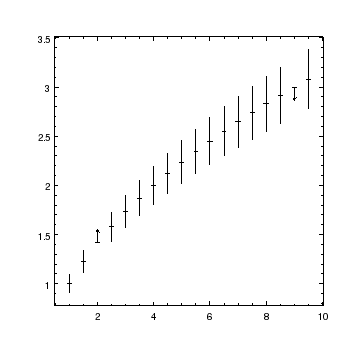### Figure 14: Y limits

The same data as shown in Figure 13 has been plotted, but this time limits are indicated for the third and last-but-one points.

Note: the limits start - rather than end - at the data point (here the a,b values).

Currently there are only two attributes that control the appearance and behavior of limits:

chips> print(get_curve().limit)
length = 0.0500000007451
override = True



Their affect can be seen in Figure 15:

chips> split(2, 1 ,0.05)
chips> add_curve (a, b, [db, db, da, da], l, ["symbol.style", "none"])
chips> set_curve(["limit.length", 0.1, "limit.override", False])


### Figure 15: Changing the appearance of limits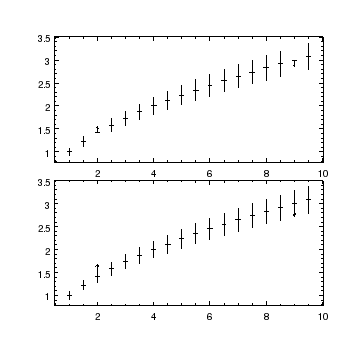### Figure 15: Changing the appearance of limits

The limit.length attribute controls the length of the line drawn to indicate the limit. The limit.override attribute determines whether the opposite error bar should be drawn (False) or not (True).

## Customizing the appearance of the axes

In this section we show some of the customizations that can be applied to axes, in particular: adjusting the position of the labels, changing the format used to draw the numeric labels, adding an extra axis to a plot, and changing the labeling of the axis.

The plot will be of the X-ray luminosity versus redshift of the clusters in the BAX database. The lx column is in units of 1044 erg/s, so we multiply by this value when creating the curve. The format for the numeric tick marks is changed for the Y axis to use the %Z format, which causes the value 1.0e44 to be written as 1 x 1044.

chips> lx = copy_colvals(cr, "lx")
chips> clear()
chips> log_scale()
chips> set_plot_xlabel("Redshift")
chips> set_plot_ylabel("L_x (erg s^{-1})")
chips> set_yaxis(["tickformat", "%Z"])


With the new label format, the label for the Y axis overlaps the ticklabels. We can, and will, increase the separation between the axis label and the axis, but first we increase the left plot margin - from 0.15 to 0.2 - to give more space.

chips> get_plot().leftmargin
0.15000000596
chips> set_plot(["leftmargin", 0.2])


There are many attributes that change the appearance of axes; Figure 16 shows the GUI created when you select Edit Axis from the right-mouse-button menu in the ChIPS window. Note that you can even re-size plots by selecting the plot and dragging the handles that appear.

### Figure 16: The attributes of an axis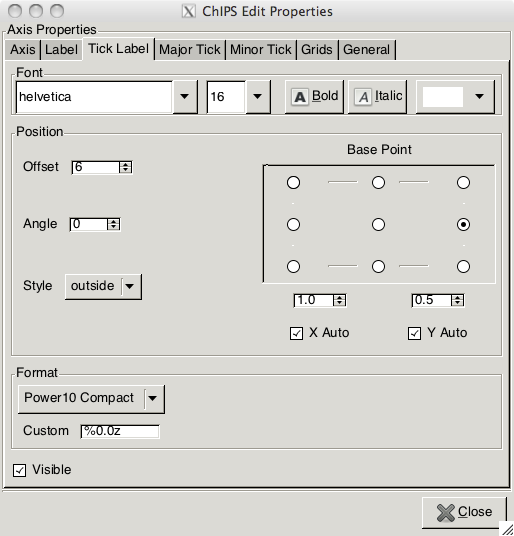### Figure 16: The attributes of an axis

Here we have selected the Y axis of a plot and used the Edit Axis menu item to bring up the Properties editor.

The tab has been changed to Tick label to concentrate on the ticklabel properties of the axis.

Here we concentrate on those that control the appearance of the labels that appear at the positions of the major tick marks on the Y axis:

chips> print(get_yaxis().ticklabel)
angle = 0.0
color = default
font = helvetica
fontstyle = normal
halign = -99.0
offset = 6
size = 12
style = outside
valign = -99.0
visible = True


We decide to increase the size of these labels, from 12 to 16, and we use the set_axis call to change both the X and Y axes at the same time. To stop the axis label from overlapping the ticklabels we increase the offset.perpendicular value from 40 to 65 (the units of this attribute is pixels).

chips> set_axis(["ticklabel.size", 16])
chips> print(get_yaxis().offset)
parallel = 0.0
perpendicular = 40.0

chips> set_yaxis(["offset.perpendicular", 65])


We now want to add an X axis to the top of the plot that indicates the luminosity distance to the clusters. We start by adding an X-axis to the top of the plot - a value of y = 1 in the PLOT_NORM coordinate system indicates the top of the plot area - and ensure that it has thee same range as the existing X axis (by use of get_plot_xrange). The pad attribute is set to 0 to make sure that these limits are not automatically expanded when the axis is created (since the default value for the attribute is 0.05), and the id attribute set to make it easier in later calls to identify which axis is which. As the new X axis is now the current X axis, the log_scale call changes the scaling of just this axis. The current state of the plot is shown in Figure 17.

chips> xr = get_plot_xrange()
chips> log_scale(X_AXIS)


### Figure 17: Adding a second X axis to the plot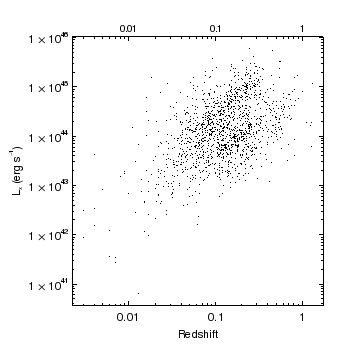### Figure 17: Adding a second X axis to the plot

An extra X axis has been added to the top of the plot. At the moment it has the same range, scaling, and labels as the bottom X axis (although the size of the ticklabels is smaller).

We now want to label the luminosity distances along this axis. We have calculated that the 100, 1000, and 10000 Mpc luminosity distances correspond to z = 0.0166, 0.16079, and 1.37052 for the cosmology used for the BAX database (an Einstein-de Sitter universe with H0 = 50 km/s/Mpc), so we can use the set_arbitrary_tick_positions call to add major tick marks at these positions. The new axis is explicitly identified in this call - i.e. the first argument is set to "dl" - otherwise the Y axis would also have been changed by this call. Since the minor tick marks have no meaning for this axis (as the scaling is neither linear or logarithmic), we turn them off by setting the minortick.visible attribute to False.

chips> xl = ["100", "1000", "10000"]
chips> zr = [0.0166, 0.16079, 1.37502]
chips> set_arbitrary_tick_positions ("dl", zr, xl)
chips> set_xaxis(["minortick.visible", False])
chips> print(info_current())
Window [win1]
Frame [frm1]
Plot [plot1]
Curve [crv1]
Y Axis [ay1]
X Axis [dl]
Coord Sys [Data]
Coord Sys ID [ds7.7.9.40]

chips> print(info())
Window [win1]
Frame [frm1]
Plot [plot1]   (0.20,0.15)  .. (0.90,0.90)
Border bottom [bx1]  top [bx2]  left [by1]  right [by2]
Curve [crv1]
X Axis [ax1]
Y Axis [ay1]
X Axis [dl]



As the new X axis is still current we can easily add a label to it and decrease the separation between the label and the new axis. To make the most use of the space we decrease the right margin of the plot from 0.1 to 0.05.

chips> set_plot_xlabel("Luminosity Distance (Mpc)")
chips> print(get_xaxis().offset)
parallel = 0.0
perpendicular = 40.0

chips> set_xaxis(["offset.perpendicular", 25])
chips> get_plot().rightmargin
0.10000000149
chips> set_plot(["rightmargin", 0.05])


Finally we print out the plot after changing the symbol to the smallest sized circle provided by ChIPS. The postscript and PDF versions are scaled up to fit a US letter page whilst keeping the aspect ratio of the plot fixed.

chips> set_yaxis(["tickformat", "%0.0z"])
chips> print_window("axes", ["fittopage", True])
chips> print_window("axes.pdf", ["fittopage", True])
chips> print_window("axes.png")
chips> print_window("axes.eps")
chips> save_state("axes.state")


### Figure 18: Customized axes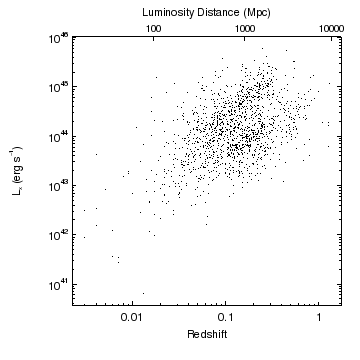### Figure 18: Customized axes

The final plot shows customizations applied to both axes. The Y axis has been changed to use scientific notation (i.e. 1042) and the label has been moved so that it no longer overlaps the ticklabels. Both the Y axis and the bottom (original) X axis have had the size of the ticklabels increased.

The axis tickformat was set to "%0.0z", which avoids displaying the leading "1 x" of exponential labels when possible, as can be seen by comparing the Y axis labels in this plot to those in Figure 17.

The second X axis, added at the top of the plot, has been adjusted so that it shows representative luminosity distance values. Since the scaling between redshift and luminosity distance is not linear or logarithmic, the minor tick marks have been hidden for this axis.

The plot margins have been adjusted so that the plot makes full use of the space it occupies, and to allow the labels on the Y axis to be moved so they do not overlap.

## Summary

• Curves can be drawn using any combination of:

1. lines connecting the points
2. symbols at the points
3. error bars at the points

Error bars can be symmetric or asymmetric and drawn for one or both axes. There is also rudimentary support for drawing upper or lower limits.

The full set of attributes for curves can be found from the preference settings:

chips> print(get_preference("curve"))
curve.stem             : crv
curve.depth            : default
curve.line.color       : default
curve.line.thickness   : 1
curve.line.style       : solid
curve.symbol.color     : default
curve.symbol.style     : cross
curve.symbol.size      : 5
curve.symbol.angle     : 0
curve.symbol.fill      : true
curve.err.color        : default
curve.err.thickness    : 1
curve.err.style        : line
curve.err.up           : on
curve.err.down         : on
curve.err.left         : on
curve.err.right        : on
curve.err.caplength    : 10
curve.limit.length     : 0.05
curve.limit.override   : true


or by using the get_curve routine (used when a curve already exists):

chips> print(get_curve())
depth = 100
err.caplength = 10
err.color = default
err.down = False
err.left = False
err.right = False
err.style = line
err.thickness = 1.0
err.up = False
id = None
limit.length = 0.0500000007451
limit.override = True
line.color = default
line.style = 0
line.thickness = 1.0
stem = None
symbol.angle = 0.0
symbol.color = default
symbol.fill = True
symbol.size = 5
symbol.style = 1

• ### Figure 19: Symbol Styles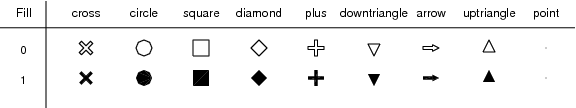### Figure 19: Symbol Styles

There are nine symbol styles that can be used (not counting the none value which turns off the symbol support for the curve). All the styles, except for the point type, can be drawn either open or filled, and at a range of sizes (1 to 100, where the default value is 5).

• Points that have one or both coordinates set to NaN are automatically ignored by ChIPS.

• The scaling used to draw an axis can be switched between linear and logarithmic using the log_scale and lin_scale routines. Values that are <=0 are ignored when an axis is drawn in a logarithmic scale.

• Annotations such as lines, labels, points, and regions, can be added to plots (Figure 6); note that they can be added directly from the GUI.

• The size and position of plots within a frame can be adjusted by changing the margin attributes of a plot - e.g. with

chips> set_plot(["leftmargin", 0.2])

or by using either of the move_plot or reposition_plot commands. The move_plot command changes the position of the plot using either relative or absolute coordinates whereas the latter (reposition_plot) can change both size and location, but only using absolute coordinates.

An alternative mechanism is to use the GUI to highlight the plot and then drag the handles around to move or re-size it.

• Regions can be filled with a color of adjustable transparency, as shown in Figure 8.

Only the PS and EPS formats do not support the alpha fill value (e.g. see the hardcopy versions of Figure 8).

If a solid fill is not required, a pattern can be used to fill regions (or histograms), as shown in Figure 10.

• The order that items are drawn is determined by the order they were created and the value of their depth attributes. This value can be adjusted, as shown when creating Figure 9, to ensure certain features are not covered up (in this case it was to avoid the filled region from blocking out the data point).

The ordering can also be changed from the GUI by selecting an object and then using the Shffle Object menu item.

• The currency settings can be used to create multiple copies of an object. For instance, to create a line at y=0 in multiple plots you can say:

chips> current_plot ("all")

• Multiple plots can be created using the split and strip_chart commands, as described in the creating and using multiple plots thread.

• Extra axes can be added to plots, as shown in Figure 17 and in the ChIPS gallery.

• Axes contain a large number of attributes to control their appearance (e.g. Figure 16). Some highlights are:

1. The formatting of ticklabels can be changed - e.g. to use scientific notation as in Figure 17.
2. The major or minor tick labels can be hidden.
3. The separation of the axis label from the axis can be changed, as in Figure 17.
4. Labels can be added at arbitrary positions for data using non-linear, or non-numeric, scales, as shown in Figure 18.

The full list of attributes can be seen in the preference settings or by calling get_xaxis or get_yaxis:

chips> print(get_preference("axis"))
axis.x.stem            : ax
axis.y.stem            : ay
axis.x.label.text      :
axis.y.label.text      :
axis.x.label.angle     : 0
axis.y.label.angle     : 90
axis.color             : default
axis.thickness         : 1
axis.depth             : default
axis.automin           : true
axis.automax           : true
axis.offset.parallel   : 0
axis.offset.perpendicular : 40
axis.tickformat        : %g
axis.ticklabel.style   : outside
axis.ticklabel.color   : default
axis.ticklabel.size    : 12
axis.ticklabel.angle   : 0
axis.ticklabel.halign  : auto
axis.ticklabel.valign  : auto
axis.ticklabel.font    : helvetica
axis.ticklabel.fontstyle : normal
axis.ticklabel.offset  : 6
axis.label.color       : default
axis.label.size        : 14
axis.label.font        : helvetica
axis.label.fontstyle   : normal
axis.label.halign      : center
axis.label.valign      : center
axis.majorgrid.visible : false
axis.majorgrid.color   : default
axis.majorgrid.style   : shortdash
axis.majorgrid.thickness : 1
axis.minorgrid.visible : false
axis.minorgrid.color   : default
axis.minorgrid.style   : dot
axis.minorgrid.thickness : 1
axis.majortick.mode    : limits
axis.majortick.interval: 10
axis.majortick.count   : 6
axis.majortick.thickness : 1
axis.majortick.length  : 4
axis.majortick.color   : default
axis.majortick.style   : inside
axis.majortick.visible : true
axis.minortick.mode    : nice
axis.minortick.interval: 5
axis.minortick.count   : 4
axis.minortick.thickness : 1
axis.minortick.length  : 2
axis.minortick.color   : default
axis.minortick.style   : inside
axis.minortick.visible : true


## History

 09 Jul 2008 New for CIAO 4.0 15 Dec 2008 CIAO 4.1 - missing values in ASCII columns are now converted to NaN rather than 0; use copy_colvals rather than get_colvals; regions can now be filled by a range of patterns (Figure 10) set_arbitrary_tick_positions now needs the "advanced" ChIPS module loaded 15 Dec 2009 Updated for CIAO 4.2: the set_arbitrary_tick_positions command has been moved back into the standard ChIPS module, the axis tickformat setting of "%0.0z" improves the display of exponential notation (Figure 18) on axes. 15 Dec 2010 Updated for CIAO 4.3 15 Dec 2011 Updated for CIAO 4.4; noted the support for alpha and opacity in PDF ouput (Figure 8); added Figure 16 to highlight the new ChIPS GUI. 13 Dec 2012 Updated for CIAO 4.5. 04 Dec 2013 Updated for CIAO 4.6. 10 Dec 2014 Updated for CIAO 4.7. 15 Dec 2015 Updated for CIAO 4.8.